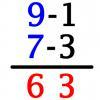#### You may also like### Triangle Incircle Iteration

Keep constructing triangles in the incircle of the previous triangle. What happens?### Vedic Sutra - All from 9 and Last from 10

Vedic Sutra is one of many ancient Indian sutras which involves a cross subtraction method. Can you give a good explanation of WHY it works?### Tournament Scheduling

Scheduling games is a little more challenging than one might desire. Here are some tournament formats that sport schedulers use.

# Zeller's Birthday

##### Age 14 to 16Challenge Level

What day of the week were you born on? Do you know?

Here's a way to find out.

For example, if your date of birth was 6 July 1989 :

$D = 6$ , $M = 7$ , and $Y = 1989$

If $M$ had been a 1 or 2, subtract 1 from $Y$ and add 12 to $M$.

$Y_F$ is made from the first two digits of $Y$ and $Y_L$ is made from the last two digits of $Y$.

To begin, work out the sum of all the integer parts of $2.6M - 5.39$ , of $\frac{Y_F}{4}$ , and of $\frac{Y_L}{4}$

Add $D$ and $Y_L$ into that total, and then subtract 2 lots of $Y_F$

Divide that final answer by 7 and notice the remainder .

A remainder value of 0 means the date was a Sunday, 1 means it was a Monday, 2 for a Tuesday, and so on.

Can you follow the method (what you actually have to do) ?

You could check some dates you happen to know the answer for ?

When you are getting good at using the method start to ask yourself how it works and why does it give the right result?

Why 2.6 and why 5.39?